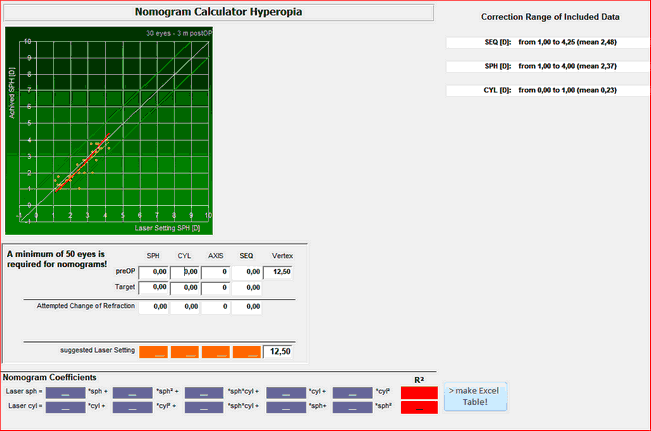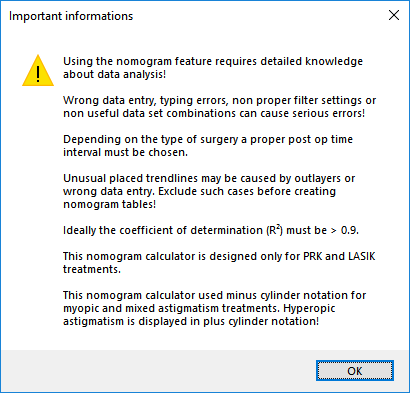﻿ Nomograms, Reports, IOL-Constants > Hyperopia Nomogram
 Navigation:  Nomograms, Reports, IOL-Constants > Hyperopia NomogramThe Hyperopia Nomogram window shows two scatter plots with green background. The left one shows laser settings for sphere on the x-Axis and the achieved spherical correction on the y-Axis. The right Scatter plot shows the corresponding laser setting for cylinder and the achieved cylinder correction in plus cylinder notation.The valid Data Range is indicated on the upper right hand side next to the scatter plots. This is the range of the achieved corrections from your current data set. Nomogram calculations will be limited to be within these boundaries.

The Nomogram Calculator allows you to enter preOP and target refractions including vertex distance and then automatically calculated the suggested laser settings for sph, cyl and axis. You may enter preOp refractions in plus or minus cylinder notation as the attempted refraction will be automatically converted to minus cylinder.

Note: suggested laser settings will always be given in minus cylinder form. You may have to convert these in case your laser asks for plus cylinder refractions!

In the lower part of the window, 2 sets of 5 Nomogram Coefficients are given, including a coefficient of determination (R²). These coefficients are the result of the multi parametric regression analysis performed by Datagraph. Suggested laser settings are calculated with these coefficients and the given formula.

R² values will have a red background in case they are below a value of 0.9, which is an indication inconsistent data or a 'bad fit'. Suggested laser settings may not be reliable if R² is below 0.9 and should not be used. The following warning will appear if at least one of the two R² coefficients is smaller than 0.9.More warning messages may appear depending on your current data selection and/or values entered as attempted change of refraction. It should be obvious that nomogram calculation can be made only for the range of data which is available and currently selected. If not, one of the following warning labels will appear.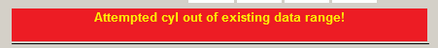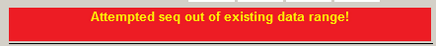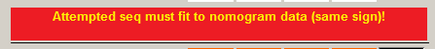To use the nomogram calculator a valid preOP and target refraction (default 0) has to be entered. Datagraph calculates the attempted change of refraction accordingly and suggested laser settings will be displayed based on the given formulas and nomogram coefficients. In case of the example below, an pre OP refaction of +3 sph / +2 cyl x 45° and a target of -1 D sph would result in a suggested laser setting of +4.59 sph and -1.68 cyl x 135°. (if your laser expects plus cylinder you will have to convert from minus to plus cylinder and enter +2.91 D sph and +1.68 D cyl x 45° in this example)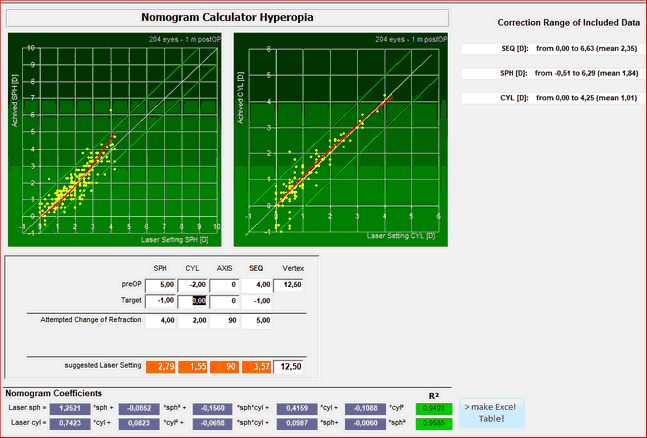The hyperopia nomogram screen may come up as 'sphere only nomogram' if the selected data set contained only spherical myopia treatments (Laser Setting Cyl = 0 in all eyes). Therefore only the left scatter plot is relevant, showing laser settings for sphere (x-axis) vs. the achieved change in sphere (y-axis).

Nomogram calculations can be made, but only for treatments of spherical myopia.

If both R² are empty and no coefficients are calculated the number of eyes in your current selection is too small. Increase to at least 50 eyes. Then coefficients will be calculated. Proceed only if R² is better than 0.9.